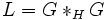Amalgam-characteristic subgroup

BEWARE! This term is nonstandard and is being used locally within the wiki. [SHOW MORE]
This article defines a subgroup property: a property that can be evaluated to true/false given a group and a subgroup thereof, invariant under subgroup equivalence. View a complete list of subgroup properties[SHOW MORE]

Definition

Definition with symbols

Suppose$G$ is a group and$H$ is a subgroup of$G$. We say that$H$ is an amalgam-characteristic subgroup of$G$ if$H$ is characteristic in the group$L$ given by:$L = G *_H G$.

In other words,$L$ is the amalgam of$G$ with itself over$H$, and$H$ is treated as the subgroup of$L$ given by the amalgamated$H$ between the two factors.

Relation with other properties

Stronger properties

Property Meaning Proof of implication Proof of strictness (reverse implication failure) Intermediate notions
finite normal subgroup normal subgroup that is finite as a group finite normal implies amalgam-characteristic Amalgam-normal-subhomomorph-containing subgroup, Amalgam-strictly characteristic subgroup, Periodic normal subgroup|FULL LIST, MORE INFO
periodic normal subgroup normal subgroup and every element has finite order periodic normal implies amalgam-characteristic Amalgam-normal-subhomomorph-containing subgroup, Amalgam-strictly characteristic subgroup|FULL LIST, MORE INFO
central subgroup contained in the center, i.e., its elements commute with every element central implies amalgam-characteristic Amalgam-strictly characteristic subgroup, Normal subgroup contained in the hypercenter|FULL LIST, MORE INFO
normal subgroup contained in the hypercenter normal and contained in the hypercenter Normal subgroup contained in hypercenter is amalgam-characteristic Amalgam-strictly characteristic subgroup|FULL LIST, MORE INFO
amalgam-normal-subhomomorph-containing subgroup normal-subhomomorph-containing subgroup in the amalgam of the whole group over it Amalgam-strictly characteristic subgroup|FULL LIST, MORE INFO
amalgam-strictly characteristic subgroup strictly characteristic subgroup in the amalgam of the whole group over it |FULL LIST, MORE INFO

Weaker properties

Property Meaning Proof of implication Proof of strictness (reverse implication failure) Intermediate notions
normal subgroup amalgam-characteristic implies normal normal not implies amalgam-characteristic |FULL LIST, MORE INFO